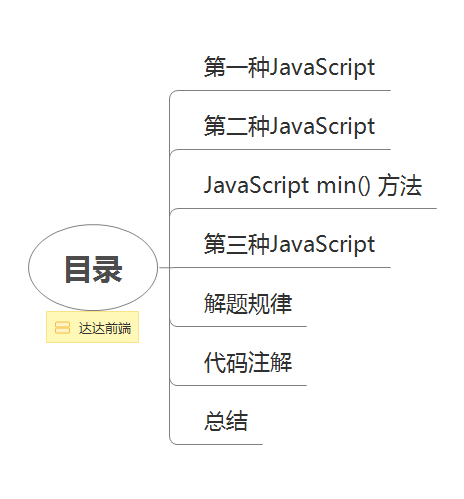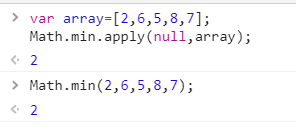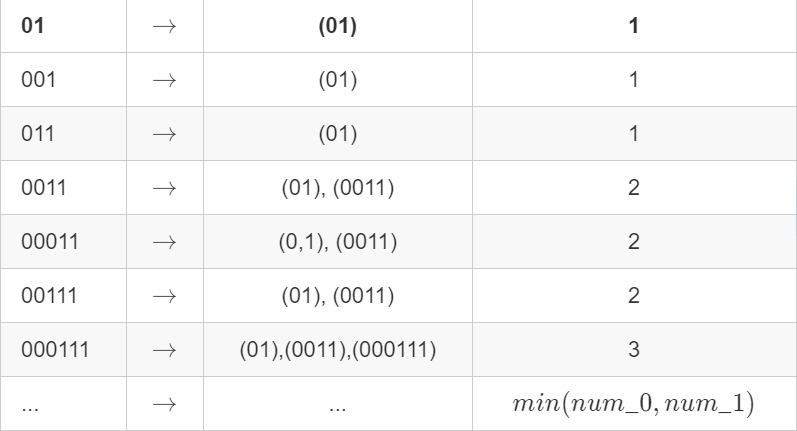## 一道二进制子串算法让面试官都解不出来？ 原# 示例1：

“0011”，“01”，“1100”，“10”，“0011”，“01”。

# 示例2：

“000111”中有多少个有效的二进制子串，“11100”中有多少个有效的二进制子串?“00011100”呢?

# JavaScript的解法：

/**

• @param {string} s
• @return {number} */ var countBinarySubstrings = function(s) {

};

# 描述：# 第一种JavaScript：

``````var countBinarySubstrings = function(s) {
// 前面一个数，和当前数
let pre=0, count=0, count1=0, count2=0;
// 循环字符串
for(let i = 1; i < s.length; i++) {
// 遇到10或01的情况，则说明连续的1或连续的0断了
if(s[i] !== s[pre]) {
if(count1 === 0) {
// 拿到前面连续1或0的数量
count1 = i - pre;
pre = i;
} else {
count2 = i - pre;
count += count1 > count2 ? count2 : count1;
count1 = count2;
count2 = 0;
pre = i;
}
}
if(i === s.length - 1) {
count2 = s.length - pre;
count += count1 > count2 ? count2 : count1;
}
}

return count;
}
``````

# 第二种JavaScript：

``````/**
* @param {string} s
* @return {number}
*/
var countBinarySubstrings = function(s) {
// 字符串的长度
const len = s.length;
// 次数为0，前一个为0，current当前为1。
let n = 0, pre = 0, current = 1;
// 循环字符串
for(let i = 0; i<len; i++){
if(s[i] === s[i+1]) {
current++;
}else {
// 比如00011,就有2组
if(pre > 0) {
n += Math.min(pre.current);
}
pre = current;
current = 1;
}
}
return n;
};
``````

# JavaScript Math 对象

min() 方法可返回指定的数字中带有最低值的数字。

Math.min.apply(null, arr)

``````var array=[2,6,5,8,7];
Math.min.apply(null,array);
``````# 第三种JavaScript：

``````/**
* @param {string} s
* @return {number}
*/
var countBinarySubstrings = function(s) {
// res 存储相邻连续字符串的个数
let res = [];
let temp = s;
let count = 0;
for(let i of s) {
// 循环字符串
if(i !== temp) {
res.push(count);
temp = i;
count = 0;
}
count++;
}
res.push(count);
let total = 0;
for(let i=0; i<res.length-1; i++){
total += Math.min(res[i], res[i+1]);
}
};
``````

``````<script type="text/javascript">

document.write(Math.min(5,7) + "<br />")
document.write(Math.min(-3,5) + "<br />")
document.write(Math.min(-3,-5) + "<br />")
document.write(Math.min(7.25,7.30))
</script>
``````

``````5
-3
-5
7.25
``````• 000111必定有三个子串
• 00011必定有两个子串
• 0111必定有1个子串

``````/**
* @param {string} s
* @return {number}
*/
var countBinarySubstrings = function(s) {
let num = 0;
const arr = s.match(/0+|1+/g);
// let n = 0, arr = s.match(/(+)|(+)/g)

// 把字符串切割成['00', '11', '00', '11']这样的数组

for(let i = 0, len = arr.length; i < len - 1; i++){
num += Math.min(arr[i].length, arr[i+1].length);
// 相邻比较，长度更短的则为这一组的出现次数
}

return num;
}
``````

# 代码注解

``````/**
* @param {string} s
* @return {number}
*/
var countBinarySubstrings = function(s) {
// 计算前一个字符连续出现的次数
let pre = 0
// 计算后一个字符连续出现的次数
let cur = 1
// 每当 pre >= cur 时，既满足条件一次 count++
// 前面有两个0，后面它自己为1
// 计数count一开始为0
let count = 0

// 循环字符串
for(let i=1; i<s.length; i++) {
// 如果前一个和后一个相等
if(s[i] === s[i-1]) {
// 本身当前它自己的数为1，那么两者相等，这个数就+1，为2
cur++
// 00
} else {
// 当出现不一样的字符时，现任变前任，现任重新计数
// 01,001,10,101,不一样的前后，10,01
// 请一个数字的数量为1，后一个数字的数量为1
pre = cur
// 01,10, 当前数还是1
cur = 1
}

// 001, 110, 010, 101,
// 只要  pre >= cur, 即可满足条件一次
if(pre >= cur) {
count++
}
}
return count
};
``````

``````// 计算前一个字符连续出现的次数
let pre = 0
// 计算后一个字符连续出现的次数
let cur = 1
// 每当 pre >= cur 时，既满足条件一次 count++
// 前面有两个0，后面它自己为1
// 计数count一开始为0
let count = 0
``````

# 总结：

## ❤️ 不要忘记留下你学习的脚印 [点赞 + 收藏 + 评论]

【作者】：Jeskson 【原创公众号】：达达前端小酒馆。 【福利】：公众号回复 “资料” 送自学资料大礼包（进群分享，想要啥就说哈，看我有没有）！ 【转载说明】：转载请说明出处，谢谢合作！~

## 请点赞！因为你们的赞同/鼓励是我写作的最大动力！

### 欢迎关注达达的CSDN！### 评论(0)

2018/06/07
0
0

2018/02/24
0
0

abcdd1234567890
2017/06/14
0
0

linux服务器架构
2019/07/22
24
0

2012/05/09
553
3

javail

19
0

honeymoose

17
0
C语言动态内存分配：（一）malloc/free的实现及malloc实际分配/释放的内存

shzwork

17
0

27
0

15
0